# spectralGraphTopology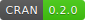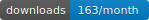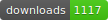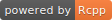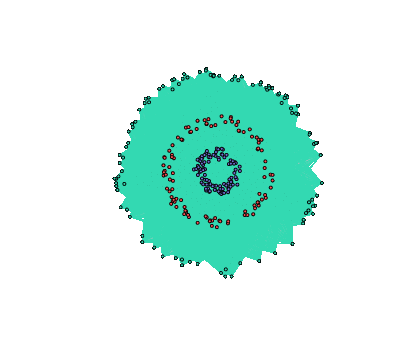spectralGraphTopology provides estimators to learn k-component, bipartite, and k-component bipartite graphs from data by imposing spectral constraints on the eigenvalues and eigenvectors of the Laplacian and adjacency matrices. Those estimators leverages spectral properties of the graphical models as a prior information, which turn out to play key roles in unsupervised machine learning tasks such as community detection.

Documentation: https://mirca.github.io/spectralGraphTopology.

## Installation

From inside an R session, type:

> install.packages("spectralGraphTopology")

Alternatively, you can install the development version from GitHub:

> devtools::install_github("dppalomar/spectralGraphTopology")

#### Microsoft Windows

On MS Windows environments, make sure to install the most recent version of Rtools.

#### macOS

spectralGraphTopology depends on RcppArmadillo which requires gfortran.

## Usage: clustering

We illustrate the usage of the package with simulated data, as follows:

library(spectralGraphTopology)
library(clusterSim)
library(igraph)
set.seed(42)

# generate graph and data
n <- 50  # number of nodes per cluster
twomoon <- clusterSim::shapes.two.moon(n)  # generate data points
k <- 2  # number of components

# estimate underlying graph
S <- crossprod(t(twomoon$data)) graph <- learn_k_component_graph(S, k = k, beta = .5, verbose = FALSE, abstol = 1e-3) # plot # build network net <- igraph::graph_from_adjacency_matrix(graph$Adjacency, mode = "undirected", weighted = TRUE)
# colorify nodes and edges
colors <- c("#706FD3", "#FF5252")
V(net)$cluster <- twomoon$clusters
E(net)$color <- apply(as.data.frame(get.edgelist(net)), 1, function(x) ifelse(V(net)$cluster[x] == V(net)$cluster[x], colors[V(net)$cluster[x]], '#000000'))
V(net)$color <- colors[twomoon$clusters]
# plot nodes
plot(net, layout = twomoon\$data, vertex.label = NA, vertex.size = 3)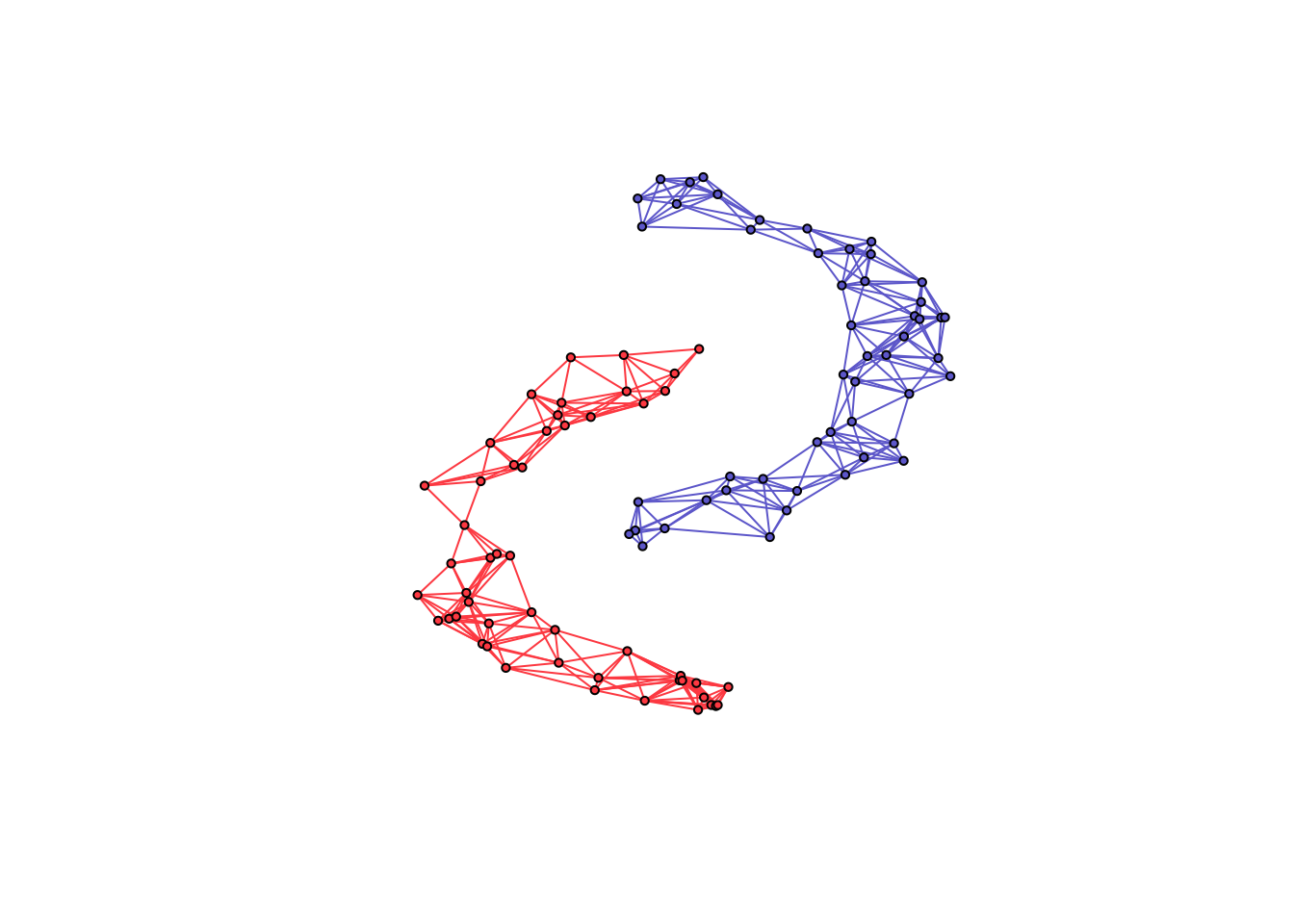## Contributing

We welcome all sorts of contributions. Please feel free to open an issue to report a bug or discuss a feature request.

## Citation

cluster_k_component_graph N., Feiping, W., Xiaoqian, J., Michael I., and H., Heng. (2016). The Constrained Laplacian Rank Algorithm for Graph-based Clustering, AAAI’16.
learn_laplacian_gle_mm Licheng Zhao, Yiwei Wang, Sandeep Kumar, and Daniel P. Palomar, Optimization Algorithms for Graph Laplacian Estimation via ADMM and MM, IEEE Trans. on Signal Processing, vol. 67, no. 16, pp. 4231-4244, Aug. 2019
learn_laplacian_gle_admm Licheng Zhao, Yiwei Wang, Sandeep Kumar, and Daniel P. Palomar, Optimization Algorithms for Graph Laplacian Estimation via ADMM and MM, IEEE Trans. on Signal Processing, vol. 67, no. 16, pp. 4231-4244, Aug. 2019
learn_combinatorial_graph_laplacian H. E. Egilmez, E. Pavez and A. Ortega, Graph learning from data under Laplacian and structural constraints, Journal of Selected Topics in Signal Processing, vol. 11, no. 6, pp. 825-841, Sept. 2017